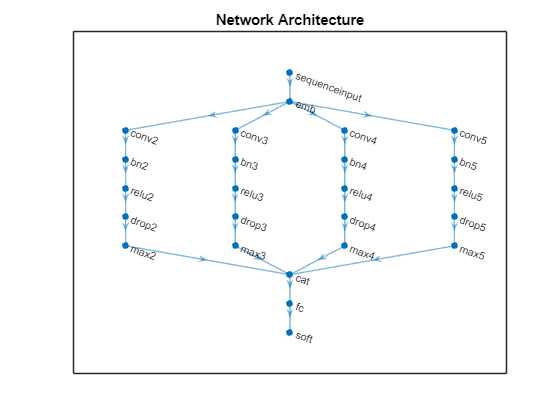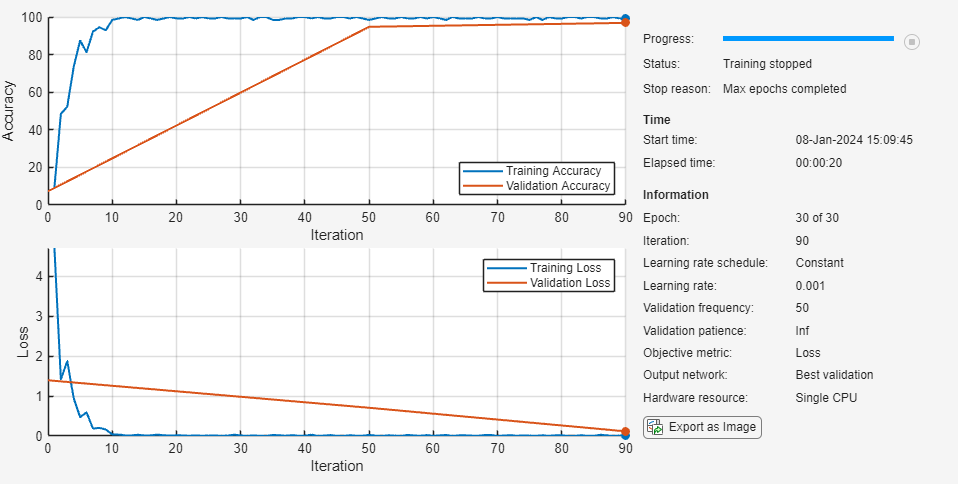Main Content

# Classify Text Data Using Convolutional Neural Network

This example shows how to classify text data using a convolutional neural network.

To classify text data using convolutions, you must convert the text data into images. To do this, pad or truncate the observations to have constant length S and convert the documents into sequences of word vectors of length C using a word embedding. You can then represent a document as a 1-by-S-by-C image (an image with height 1, width S, and C channels).

To convert text data from a CSV file to images, create a `tabularTextDatastore` object. The convert the data read from the `tabularTextDatastore` object to images for deep learning by calling `transform` with a custom transformation function. The `transformTextData` function, listed at the end of the example, takes data read from the datastore and a pretrained word embedding, and converts each observation to an array of word vectors.

This example trains a network with 1-D convolutional filters of varying widths. The width of each filter corresponds the number of words the filter can see (the n-gram length). The network has multiple branches of convolutional layers, so it can use different n-gram lengths.

### Load Pretrained Word Embedding

Load the pretrained fastText word embedding. This function requires the Text Analytics Toolbox™ Model for fastText English 16 Billion Token Word Embedding support package. If this support package is not installed, then the function provides a download link.

`emb = fastTextWordEmbedding;`

### Load Data

Create a tabular text datastore from the data in `factoryReports.csv`. Read the data from the `"Description"` and `"Category"` columns only.

```filenameTrain = "factoryReports.csv"; textName = "Description"; labelName = "Category"; ttdsTrain = tabularTextDatastore(filenameTrain,'SelectedVariableNames',[textName labelName]);```

Preview the datastore.

```ttdsTrain.ReadSize = 8; preview(ttdsTrain)```
```ans=8×2 table Description Category _______________________________________________________________________ ______________________ {'Items are occasionally getting stuck in the scanner spools.' } {'Mechanical Failure'} {'Loud rattling and banging sounds are coming from assembler pistons.'} {'Mechanical Failure'} {'There are cuts to the power when starting the plant.' } {'Electronic Failure'} {'Fried capacitors in the assembler.' } {'Electronic Failure'} {'Mixer tripped the fuses.' } {'Electronic Failure'} {'Burst pipe in the constructing agent is spraying coolant.' } {'Leak' } {'A fuse is blown in the mixer.' } {'Electronic Failure'} {'Things continue to tumble off of the belt.' } {'Mechanical Failure'} ```

Create a custom transform function that converts data read from the datastore to a table containing the predictors and the responses. The `transformTextData` function, listed at the end of the example, takes the data read from a `tabularTextDatastore` object and returns a table of predictors and responses. The predictors are 1-by-`sequenceLength`-by-C arrays of word vectors given by the word embedding `emb`, where C is the embedding dimension. The responses are categorical labels over the classes in `classNames`.

Read the labels from the training data using the `readLabels` function, listed at the end of the example, and find the unique class names.

```labels = readLabels(ttdsTrain,labelName); classNames = unique(labels); numObservations = numel(labels);```

Transform the datastore using `transformTextData` function and specify a sequence length of 14.

```sequenceLength = 14; tdsTrain = transform(ttdsTrain, @(data) transformTextData(data,sequenceLength,emb,classNames))```
```tdsTrain = TransformedDatastore with properties: UnderlyingDatastore: [1×1 matlab.io.datastore.TabularTextDatastore] SupportedOutputFormats: ["txt" "csv" "xlsx" "xls" "parquet" "parq" "png" "jpg" "jpeg" "tif" "tiff" "wav" "flac" "ogg" "mp4" "m4a"] Transforms: {@(data)transformTextData(data,sequenceLength,emb,classNames)} IncludeInfo: 0 ```

Preview the transformed datastore. The predictors are 1-by-S-by-C arrays, where S is the sequence length and C is the number of features (the embedding dimension). The responses are the categorical labels.

`preview(tdsTrain)`
```ans=8×2 table Predictors Responses _________________ __________________ {1×14×300 single} Mechanical Failure {1×14×300 single} Mechanical Failure {1×14×300 single} Electronic Failure {1×14×300 single} Electronic Failure {1×14×300 single} Electronic Failure {1×14×300 single} Leak {1×14×300 single} Electronic Failure {1×14×300 single} Mechanical Failure ```

### Define Network Architecture

Define the network architecture for the classification task.

The following steps describe the network architecture.

• Specify an input size of 1-by-S-by-C, where S is the sequence length and C is the number of features (the embedding dimension).

• For the n-gram lengths 2, 3, 4, and 5, create blocks of layers containing a convolutional layer, a batch normalization layer, a ReLU layer, a dropout layer, and a max pooling layer.

• For each block, specify 200 convolutional filters of size 1-by-N and pooling regions of size 1-by-S, where N is the n-gram length.

• Connect the input layer to each block and concatenate the outputs of the blocks using a depth concatenation layer.

• To classify the outputs, include a fully connected layer with output size K, a softmax layer, and a classification layer, where K is the number of classes.

First, in a layer array, specify the input layer, the first block for unigrams, the depth concatenation layer, the fully connected layer, the softmax layer, and the classification layer.

```numFeatures = emb.Dimension; inputSize = [1 sequenceLength numFeatures]; numFilters = 200; ngramLengths = [2 3 4 5]; numBlocks = numel(ngramLengths); numClasses = numel(classNames);```

Create a layer graph containing the input layer. Set the normalization option to `'none'` and the layer name to `'input'`.

```layer = imageInputLayer(inputSize,'Normalization','none','Name','input'); lgraph = layerGraph(layer);```

For each of the n-gram lengths, create a block of convolution, batch normalization, ReLU, dropout, and max pooling layers. Connect each block to the input layer.

```for j = 1:numBlocks N = ngramLengths(j); block = [ convolution2dLayer([1 N],numFilters,'Name',"conv"+N,'Padding','same') batchNormalizationLayer('Name',"bn"+N) reluLayer('Name',"relu"+N) dropoutLayer(0.2,'Name',"drop"+N) maxPooling2dLayer([1 sequenceLength],'Name',"max"+N)]; lgraph = addLayers(lgraph,block); lgraph = connectLayers(lgraph,'input',"conv"+N); end```

View the network architecture in a plot.

```figure plot(lgraph) title("Network Architecture")```Add the depth concatenation layer, the fully connected layer, the softmax layer, and the classification layer.

```layers = [ depthConcatenationLayer(numBlocks,'Name','depth') fullyConnectedLayer(numClasses,'Name','fc') softmaxLayer('Name','soft') classificationLayer('Name','classification')]; lgraph = addLayers(lgraph,layers); figure plot(lgraph) title("Network Architecture")```Connect the max pooling layers to the depth concatenation layer and view the final network architecture in a plot.

```for j = 1:numBlocks N = ngramLengths(j); lgraph = connectLayers(lgraph,"max"+N,"depth/in"+j); end figure plot(lgraph) title("Network Architecture")```### Train Network

Specify the training options:

• Train with a mini-batch size of 128.

• Do not shuffle the data because the datastore is not shuffleable.

• Display the training progress plot and suppress the verbose output.

```miniBatchSize = 128; numIterationsPerEpoch = floor(numObservations/miniBatchSize); options = trainingOptions('adam', ... 'MiniBatchSize',miniBatchSize, ... 'Shuffle','never', ... 'Plots','training-progress', ... 'Verbose',false);```

Train the network using the `trainNetwork` function.

`net = trainNetwork(tdsTrain,lgraph,options);`### Predict Using New Data

Classify the event type of three new reports. Create a string array containing the new reports.

```reportsNew = [ "Coolant is pooling underneath sorter." "Sorter blows fuses at start up." "There are some very loud rattling sounds coming from the assembler."];```

Preprocess the text data using the preprocessing steps as the training documents.

`XNew = preprocessText(reportsNew,sequenceLength,emb);`

Classify the new sequences using the trained LSTM network.

`labelsNew = classify(net,XNew)`
```labelsNew = 3×1 categorical Leak Electronic Failure Mechanical Failure ```

### Read Labels Function

The `readLabels` function creates a copy of the `tabularTextDatastore` object `ttds` and reads the labels from the `labelName` column.

```function labels = readLabels(ttds,labelName) ttdsNew = copy(ttds); ttdsNew.SelectedVariableNames = labelName; tbl = readall(ttdsNew); labels = tbl.(labelName); end```

### Transform Text Data Function

The `transformTextData` function takes the data read from a `tabularTextDatastore` object and returns a table of predictors and responses. The predictors are 1-by-`sequenceLength`-by-C arrays of word vectors given by the word embedding `emb`, where C is the embedding dimension. The responses are categorical labels over the classes in `classNames`.

```function dataTransformed = transformTextData(data,sequenceLength,emb,classNames) % Preprocess documents. textData = data{:,1}; % Prepocess text dataTransformed = preprocessText(textData,sequenceLength,emb); % Read labels. labels = data{:,2}; responses = categorical(labels,classNames); % Convert data to table. dataTransformed.Responses = responses; end```

### Preprocess Text Function

The `preprocessTextData` function takes text data, a sequence length, and a word embedding and performs these steps:

1. Tokenize the text.

2. Convert the text to lowercase.

3. Converts the documents to sequences of word vectors of the specified length using the embedding.

4. Reshapes the word vector sequences to input into the network.

```function tbl = preprocessText(textData,sequenceLength,emb) documents = tokenizedDocument(textData); documents = lower(documents); % Convert documents to embeddingDimension-by-sequenceLength-by-1 images. predictors = doc2sequence(emb,documents,'Length',sequenceLength); % Reshape images to be of size 1-by-sequenceLength-embeddingDimension. predictors = cellfun(@(X) permute(X,[3 2 1]),predictors,'UniformOutput',false); tbl = table; tbl.Predictors = predictors; end```

## See Also

| | | | | | (Text Analytics Toolbox) | (Text Analytics Toolbox) | (Text Analytics Toolbox) | (Text Analytics Toolbox) | (Text Analytics Toolbox)

Download ebook